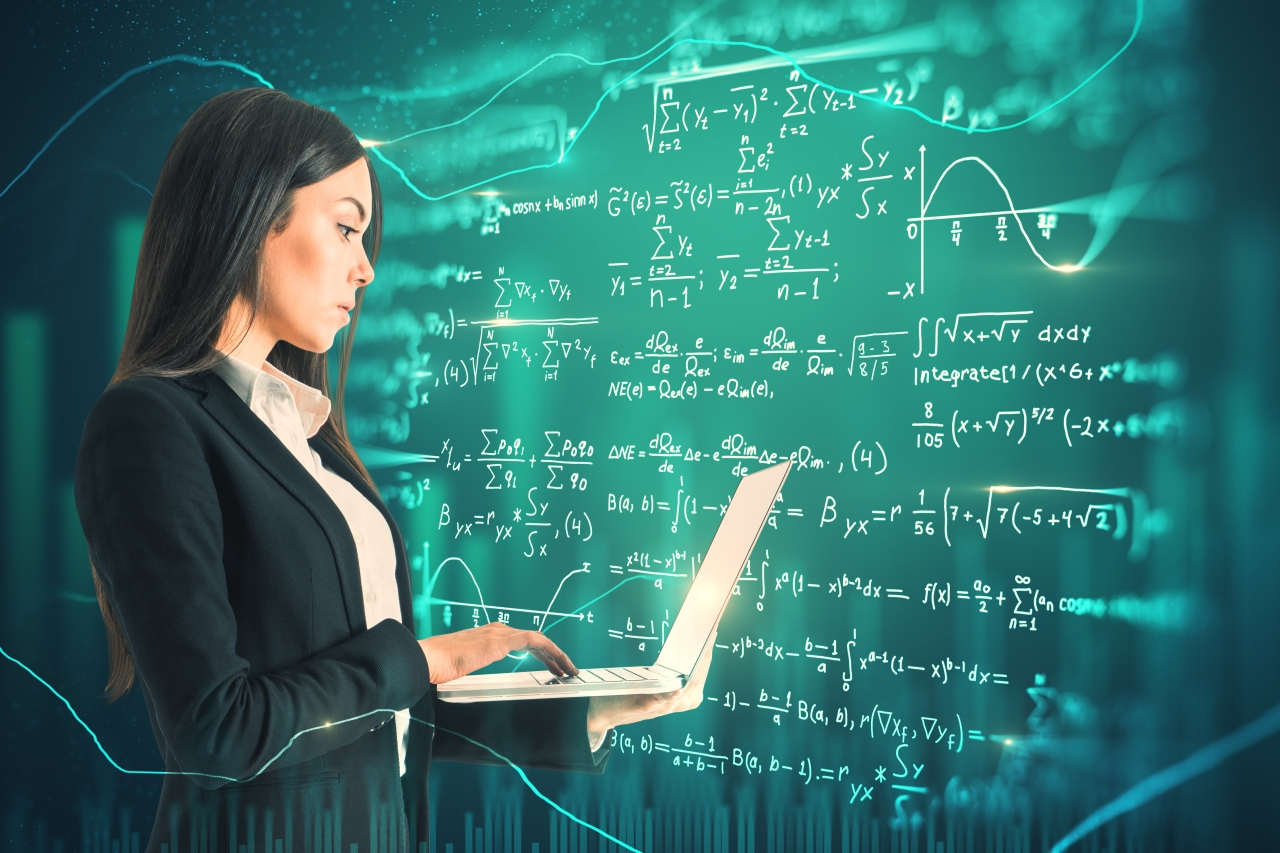# Top 10 Machine Learning Algorithms for Beginners to Dive IntoAll machine learning fields—Supervised, Unsupervised, Semi-supervised, and Reinforcement learning, use several algorithms for different types of tasks like prediction, classification, regression, etc. Each machine learning algorithm handles one specific problem, and this way beginners can dive into one of these to figure out solutions, one at a time.

Here is a compilation of the top machine learning algorithms that are frequently used in all machine learning fields.

Now, you can practice ML algorithms here.

Forming relationships between two variables is almost the starting point of a model, and linear regression in machine learning achieves that. The relationship between the dependent and independent variables is established by aligning them on a regression line. Then, the objective is to find the best fit line that explains the relationship between both variables.

The linear regression line is represented by a mathematical equation by,

Where y is the dependent variable, x is the independent variable, m is the slope, and c is the intercept.

Now, when the dependent variable is dichotomous (binary), logistic regression is used to estimate the discrete values (unlike linear regression that handles continuous values) within a set of independent variables.

This algorithm is used in predictive analysis where probability of an event occurrence is predicted based on logit function, which is why it is also called ‘logit regression’.

Mathematically, it is represented by,

Where x is the input value, y is the predicted output, b0 is the bias, and b1 is the coefficient for x.

ANNs are used in most of the recent AGI-related models that use self-supervised learning. This algorithm tries to mimic the human brain by copying the behaviour and connections of the neurons. The structure of ANNs has three or more layers that are interconnected for processing input data.

These are used in various smart appliances as well as automation devices like automatic cars, smart speakers and lights, and much more.

Convolutional neural networks (CNNs), one of the most used neural networks in recent developments, are a type of ANNs. These are mostly computer vision-based networks where the first layer is the input layer, the layers in between are the hidden layers that do that computing, and the third layer is the output layer.

An optimisation algorithm for minimising cost function by updating parameters of the machine learning model. It is used inside various machine learning algorithms and is most commonly used in deep learning. It is used in various fields like robotics, computer games, mechanical engineering, and more.

There are three types of gradient descent algorithms:

A supervised learning algorithm used for visualisation of a map of possible results for a series of decisions. Basically, it splits the dataset into two or more homogeneous for comparison of possible outcomes and then makes decisions based on advantages and probabilities.

It is like making a pros and cons list, and making decisions based on anticipations and potentiality of different options but, in machine learning, it is based on a mathematical construct.

Bayesian probability is a type of probability concept where instead of frequency of a phenomenon, probability is interpreted by quantification of a personal belief or knowledge representing a reasonable expectation. The Naive Bayes is used for classification problems, and it assumes that features in the algorithm are independent of each other and are not impacted by changes in each other.

For example, the weight and size of a table can change and maybe interrelated but do not change the fundamental fact that it is a table. This simplistic algorithm is capable of handling large datasets and making predictions in real-time.

Bayes’ theorem is given by,

Where P(X) is the probability of X being true, P(X/Y) is the conditional probability where X is true when Y is true as well.

This supervised machine learning algorithm classifies all new cases based on old cases stored that are segregated into different classes based on their similarity scores. K Nearest Neighbours (KNN) is used for both regression and classification problems.

K refers to the number of nearby points considered during segregation and classification of a set of known groups. The algorithm does classification by a majority vote of the neighbouring K points.

Major use cases and real-life applications of the algorithm can be found in recommendation systems of OTT platforms like Amazon and Netflix, and also facial recognition systems.

For clustering tasks, K-means is an unsupervised machine learning algorithm based on distance. The algorithm classifies datasets into K clusters where within one set, the data points remain homogenous, but not in different clusters.

This algorithm is used in clustering Facebook users who have common interests based on their likes and dislikes, and also segmentation of similar eCommerce products.

Another supervised learning algorithm, Random trees is a collection of multiple decision trees that are built on different samples during training. It builds on the accuracy of decision trees by mapping decisions from different trees onto a single tree known as a CART model (Classification and Regression Trees).

This helps in increasing accuracy when in a dataset, a large chunk of data is missing. The final prediction is based on the prediction result which is voted the highest. This algorithm is mostly used in eCommerce recommendation engines and financial models.

SVMs are supervised machine learning algorithms that plot individual data into a number of dimensional spaces, based on the number of features. Classification is performed by determining the hyper-plane that distincts two sets of support vectors.

Simply, SVMs are for representing coordinates of individual observations. These are popularly used in machine learning applications like facial expression classification, speech recognition, and image detection.

Images Powered by